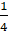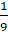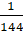Daily Practice Problems
Class 7 Maths
Fractions and DecimalsQuestion 1:

1.(a) Like fractions

(b) Irreducible fractions

(c) Equivalent fractions

(d) None of these

2. 36 ÷=?

(a) 9

(b)(c)(d) 144

Question 2:

Express 8 cm in metre and Kilometre.

Question 3:

By how much should 32.67 be increased to get 60.1?

Question 4:

A tin contains 18 Kg ghee. After consumingof it, how much ghee left in the tin?

Question 5:

Diameter of Earth is 12756000 m. In 1996, a new planet was discovered whose diameter isof the diameter of Earth. Find the diameter of this planet in km.

Question 6:

Simplify:     63.7 – 28.89 + 76.4 -37.66

Question 7:

A floor is 4.5 m long and 3.6 m wide. A 6 cm square tile costs ₹ 23.25. What will be the cost to cover the floor with these tiles?

Question 8:

Seema and Khushi want to make dresses for their dolls. Seema hasm of cloth, and she gaveof it to Khushi. How much did Khushi have?

Question 9:Question 10:

The time taken by Rohan in five different races to run a distance of 500 m was 3.20 minutes, 3.37 minutes, 3.29 minutes, 3.17 minutes and 3.32 minutes. Find the average time taken by him in the races?

Question 11:

Find the product:

(i) 67.24 X 10

(ii) 4.956 X 100

(iii) 2.3748 X 1000

Question 12:Question 13:

Arrange the following fractions in ascending order:Question 14:

A bus can cover 62.5 km in one hour. How much distance can it cover in 18 hours?

Question 15:

Describe two methods to compareand 0.86. Which do you think is easier and why?

Question 16:

Reemu read theth pages of a book. If she reads further 40 pages, she would have readth pages of the book. How many pages are left to be read?

Question 17:

A cost of pen is Rs.and that of a pencil is Rs.. Which costs more and by how much?

Question 18:

Subtract 47.56 from 83.2.

Question 19:

Express in Kg using decimals:

(a) 60 g

(b) 6 Kg 8 g

Question 20:

Convertinto irreducible form.

**********